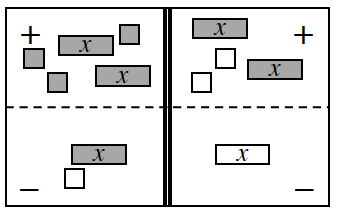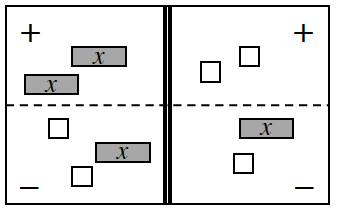### Home > INT1 > Chapter A > Lesson A.1.7 > ProblemA-71

A-71.

1.The dark boxes are positive, and the white boxes are negative.
Watch out for subtraction errors when completing this problem.

1.The dark boxes are positive, and the white boxes are negative.
Watch out for subtraction errors when completing this problem.

1. $3c−7=−c+1$

Assume that $c = x$.

$c = 2$

1. $−2+3x=2x+6+x$

no solution